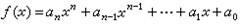﻿﻿﻿ 程序员面试之经典基础算法面试题 - TOMMYHU - 专注互联网开发及运营技术，提供相关资料及软件下载，奇趣网络时事评论！
Aug 12

#### 程序员面试之经典基础算法面试题tommyhu , 11:59 , ASP.NET , Comments(0) , Trackbacks(0) , Reads(5649) , Via OriginalLarge | Medium | Small程序员面试之经典基础算法面试题

## 1. 打靶问题的递归解法

+ 第10枪打3环，剩下9枪打87环的可能 + 第10枪打4环，剩下9枪打86环的可能 + 第10枪打5环，剩下9枪打85环的可能
+ 第10枪打6环，剩下9枪打84环的可能 + 第10枪打7环，剩下9枪打83环的可能 + 第10枪打8环，剩下9枪打82环的可能
+ 第10枪打9环，剩下9枪打81环的可能 + 第10枪打10环，剩下9枪打80环的可能。1. 若环数小于0 或者 剩下的环数大于剩下的枪数乘以10（即剩下每枪打10环用不玩所剩环数），则该递归路径不记入可能情况。
2. 若不满足条件1，且所剩枪数为1，则该递归路径记为1中可能情况。

` 1:  #include <stdio.h>`
` 2:  `
` 3:  int recursion(int count, int score)`
` 4:  {`
` 5:      if (score < 0 || score > count * 10) {`
` 6:          return 0;`
` 7:      }`
` 8:   `
` 9:      if (1 ==  count) {`
`10:          return 1;`
`11:      }    `
`12:   `
`13:      int i = 0;`
`14:      int sum = 0;`
`15:   `
`16:      for (; i <= 10; ++i) {`
`17:          sum += recursion(count - 1, score - i);`
`18:      }`
`19:   `
`20:      return sum;`
`21:  }`
`22:   `
`23:  int main()`
`24:  {`
`25:      printf("result: %d\n", recursion(10, 90));        `
`26:      return 0;`
`27:  }`

## 2. 求多项式的值与求幂的快速算法

### 2.1 求多项式的值` 1:  #include <stdio.h>`
` 2:  `
` 3:  int polynomial(const int *coefficient, int n, int x)`
` 4:  {`
` 5:      int sum = 0;`
` 6:   `
` 7:      int i = n - 1;`
` 8:      for (; i != 0; --i) {`
` 9:          sum = sum * x + coefficient[i];`
`10:      }`
`11:   `
`12:      return sum * x + coefficient;`
`13:  }`
`14:   `
`15:  int main()`
`16:  {`
`17:      int coeff = {1, 2, 3, 4, 5};`
`18:      printf("result: %d\n", polynomial(coeff, sizeof(coeff) / sizeof(coeff), 10));`
`19:      return 0;`
`20:  }`

### 2.2 求幂的快速算法

` 1:  #include <stdio.h>`
` 2:  `
` 3:  /* 统计乘法的次数 */`
` 4:  static int multiply_count = 0;`
` 5:   `
` 6:  /* 求x的p次幂 */`
` 7:  int power(int x, int p)`
` 8:  {`
` 9:      int ret =  0;`
`10:   `
`11:      if (1 == p) {`
`12:          return x;`
`13:      }`
`14:   `
`15:      /* 先计算x的p/2次幂 */`
`16:  ret = power(x, p >> 1);`
`17:      ret *= ret;`
`18:      ++multiply_count;`
`19:   `
`20:      /* 若p是奇数，则再乘一次x */`
`21:  if (0 != p % 2 /* 求模本身是一个耗性能的运算，这里可优化为 p – ((p >> 1) << 1) */) { `
`22:          ret *= x;`
`23:          ++multiply_count;`
`24:      }    `
`25:   `
`26:      return ret;`
`27:  }`
`28:   `
`29:  int main()`
`30:  {`
`31:      printf("resulut: %d\n", power(2, 20));    `
`32:      printf("multiply count: %d\n", multiply_count);`
`33:      return 0;`
`34:  }`

1. 对于需要高频率计算幂的情况；
2. 对于大数高精度计算的情况，如需计算2的10000次幂。

## 3. 一年中的第n天是几月几号？

1. 给定某年某月某日，问这是这年的第多少天？
2. 已知某年的1月1号是星期几，求给定的某年某月某日是星期几？

#define IS_LEAP(X) (((X) % 400 == 0 || (X) % 100 != 0 && (X) % 4 == 0) ? 1 : 0)

int day_count_of_month = {

{31, 28, 31, 30, 31, 30, 31, 31, 30, 31, 30, 31},
{31, 29, 31, 30, 31, 30, 31, 31, 30, 31, 30, 31}

};

` 1:  #include <stdio.h> `
` 2:  `
` 3:  #define IS_LEAP(X) (((X) % 400 == 0 || (X) % 100 != 0 && (X) % 4 == 0) ? 1 : 0)`
` 4:  int day_count_of_month = {{31, 28, 31, 30, 31, 30, 31, 31, 30, 31, 30, 31}, `
` 5:                                   {31, 29, 31, 30, 31, 30, 31, 31, 30, 31, 30, 31} }; `
` 6:  /* 计算year年的第n天是几月几号 */`
` 7:  void convert_to_date(int year, int n) `
` 8:  { `
` 9:      int leap = IS_LEAP(year); `
`10:      if(year < 1970 || year > 5000 || n <= 0 || n > 365 + leap) { `
`11:          printf("bad year\n"); `
`12:      }`
`13:   `
`14:      int i = 0; `
`15:      for(; n > day_count_of_month[leap][i]; ++i) { `
`16:          n -= day_count_of_month[leap][i]; `
`17:      } `
`18:   `
`19:      printf("year : %d, month : %d, day : %d\n", year, i + 1, n); `
`20:  } `
`21:   `
`22:  int main() `
`23:  { `
`24:      convert_to_date(2012, 230); `
`25:  } `加州求职记（转）新宇软件面试题
•中查看更多“程序员面试之经典基础算法面试题”相关内容
•中查看更多“程序员面试之经典基础算法面试题”相关内容
•中查看更多“程序员面试之经典基础算法面试题”相关内容
•中查看更多“程序员面试之经典基础算法面试题”相关内容